Скачать презентацию Note for teachers This worked well as a

ffd17f131a8ced808bb9c505bd5634d3.ppt

• Количество слайдов: 61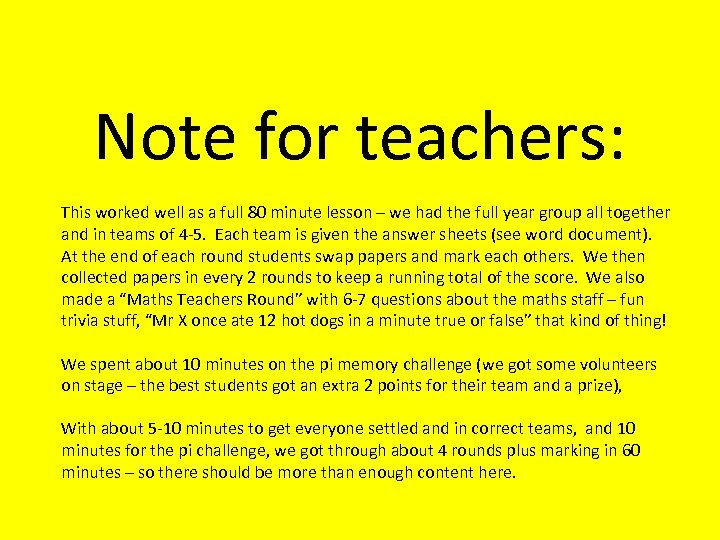Note for teachers: This worked well as a full 80 minute lesson – we had the full year group all together and in teams of 4 -5. Each team is given the answer sheets (see word document). At the end of each round students swap papers and mark each others. We then collected papers in every 2 rounds to keep a running total of the score. We also made a “Maths Teachers Round” with 6 -7 questions about the maths staff – fun trivia stuff, “Mr X once ate 12 hot dogs in a minute true or false” that kind of thing! We spent about 10 minutes on the pi memory challenge (we got some volunteers on stage – the best students got an extra 2 points for their team and a prize), With about 5 -10 minutes to get everyone settled and in correct teams, and 10 minutes for the pi challenge, we got through about 4 rounds plus marking in 60 minutes – so there should be more than enough content here.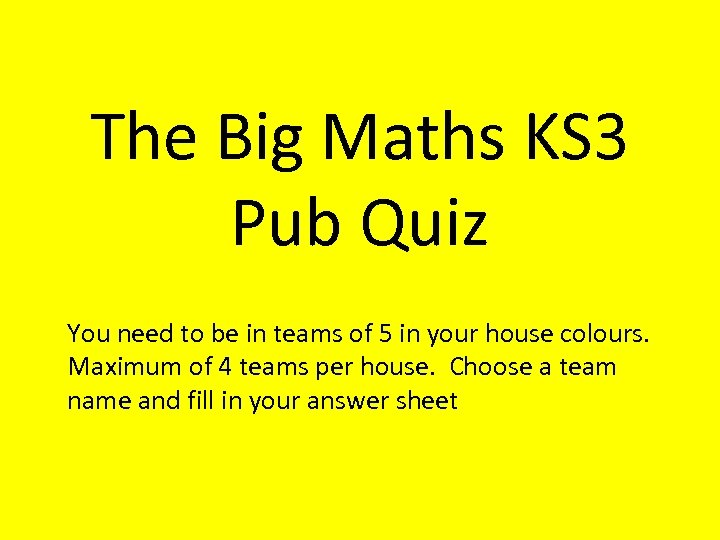The Big Maths KS 3 Pub Quiz You need to be in teams of 5 in your house colours. Maximum of 4 teams per house. Choose a team name and fill in your answer sheet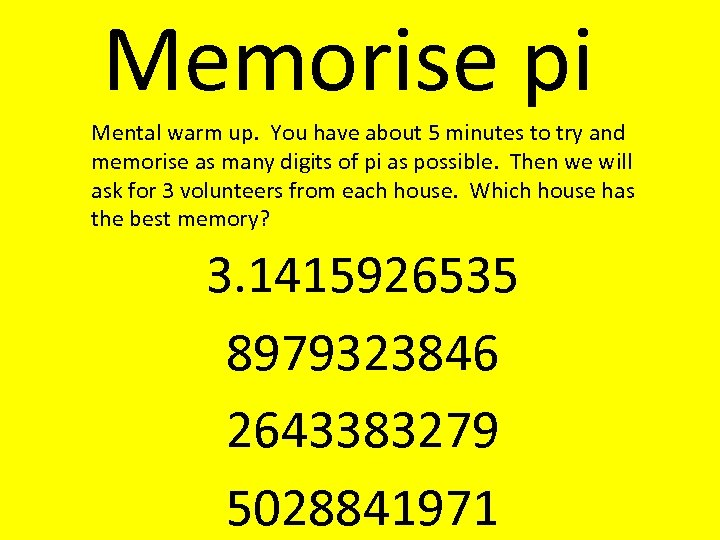Memorise pi Mental warm up. You have about 5 minutes to try and memorise as many digits of pi as possible. Then we will ask for 3 volunteers from each house. Which house has the best memory? 3. 1415926535 8979323846 2643383279 5028841971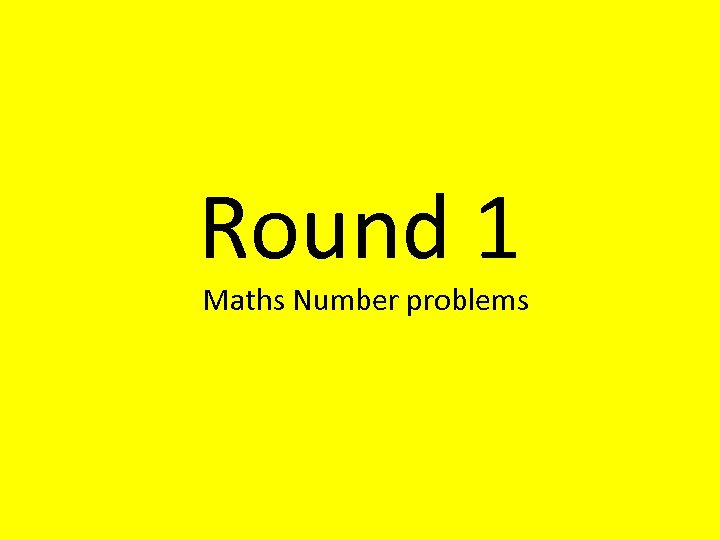Round 1 Maths Number problems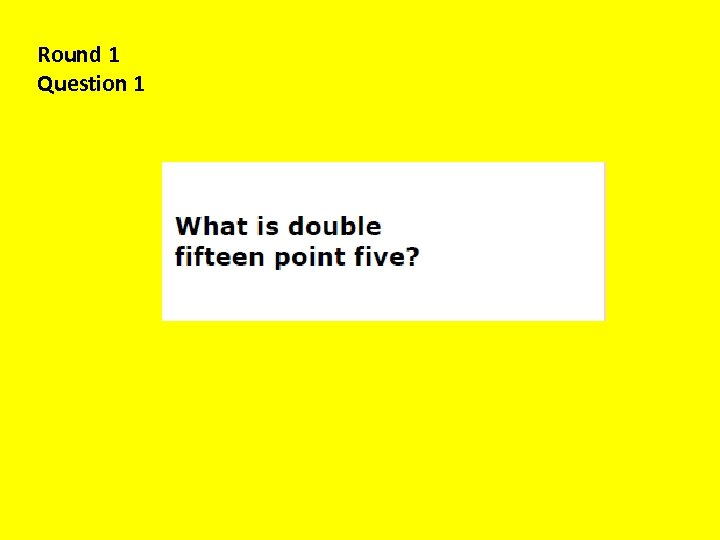Round 1 Question 1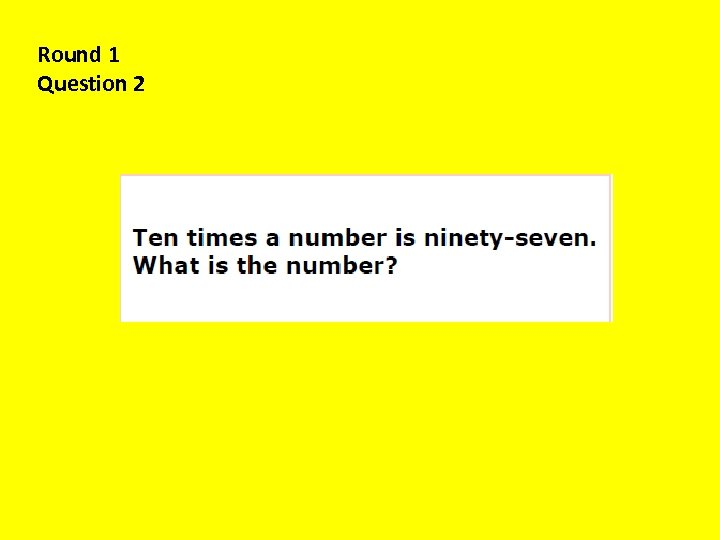Round 1 Question 2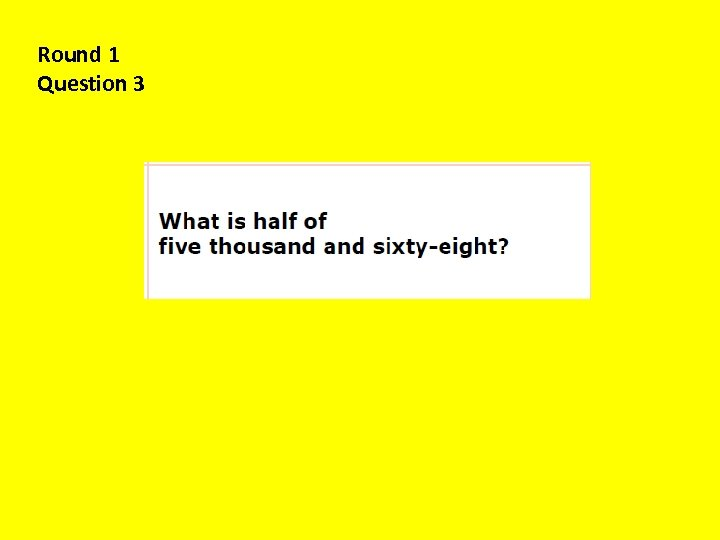Round 1 Question 3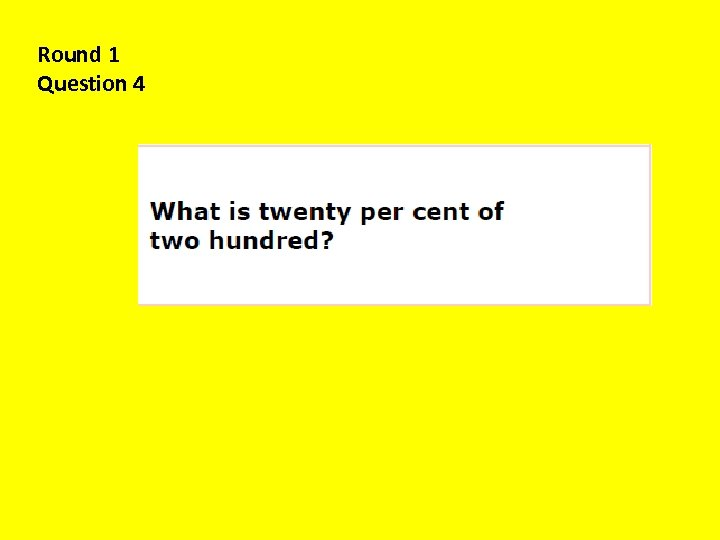Round 1 Question 4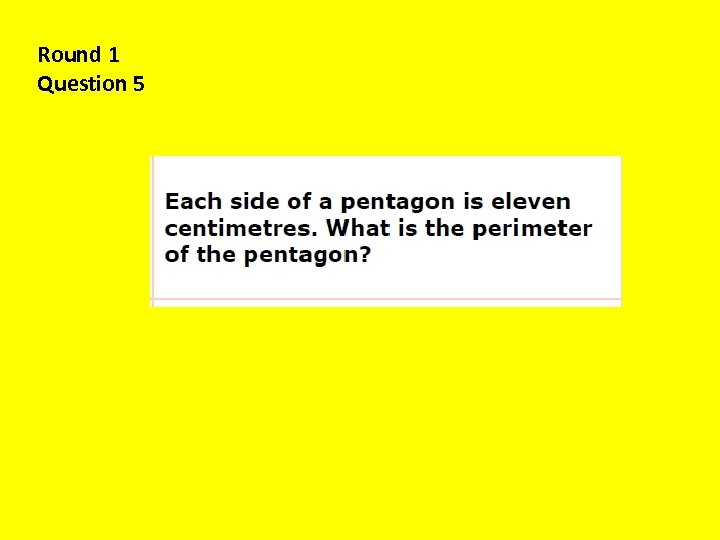Round 1 Question 5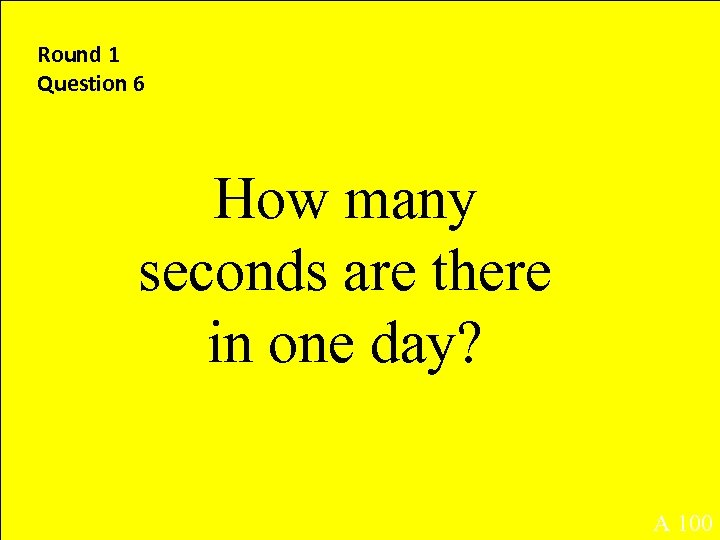Round 1 Question 6 How many seconds are there in one day? A 100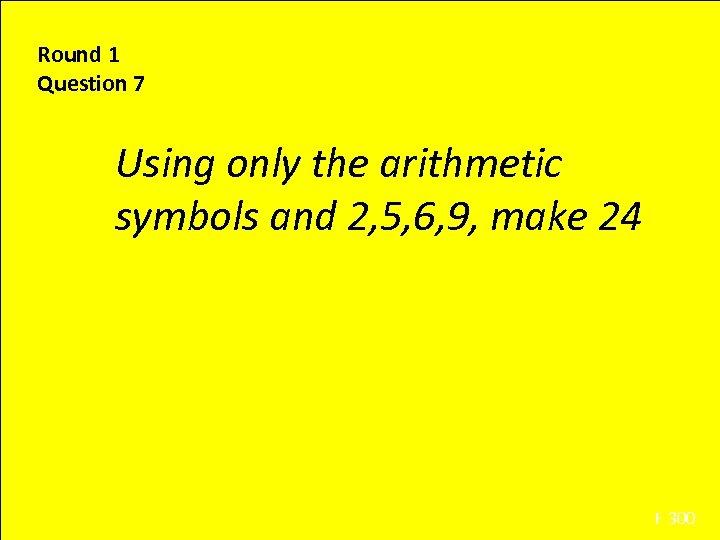Round 1 Question 7 Using only the arithmetic symbols and 2, 5, 6, 9, make 24 F 300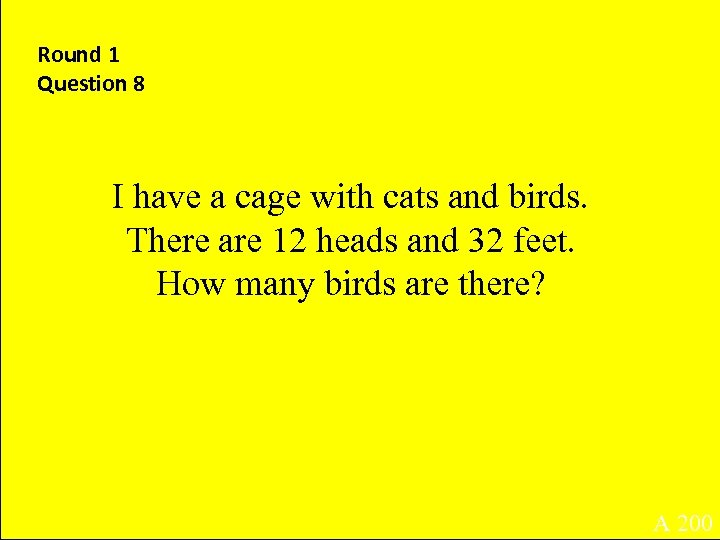Round 1 Question 8 I have a cage with cats and birds. There are 12 heads and 32 feet. How many birds are there? A 200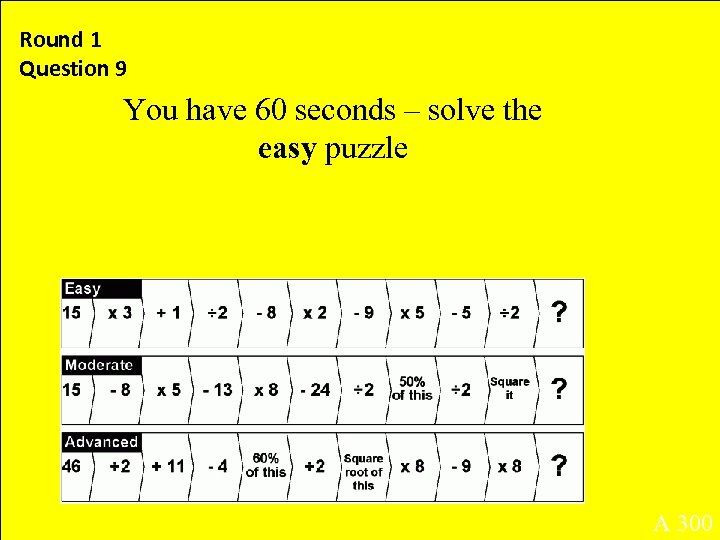Round 1 Question 9 You have 60 seconds – solve the easy puzzle A 300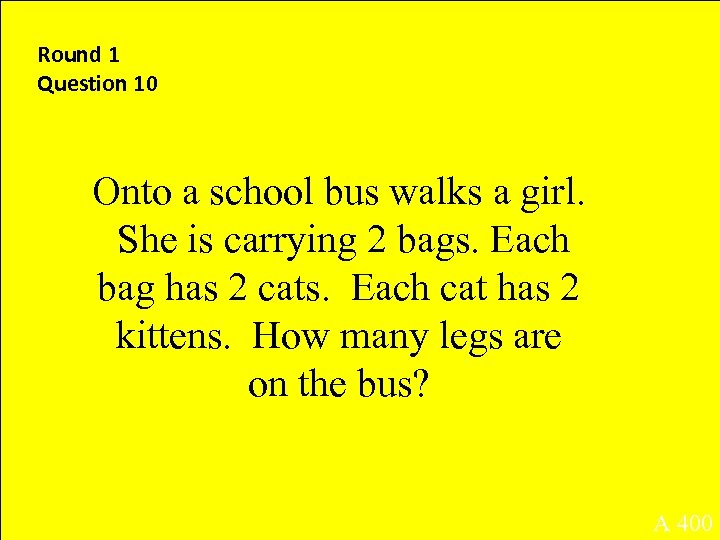Round 1 Question 10 Onto a school bus walks a girl. She is carrying 2 bags. Each bag has 2 cats. Each cat has 2 kittens. How many legs are on the bus? A 400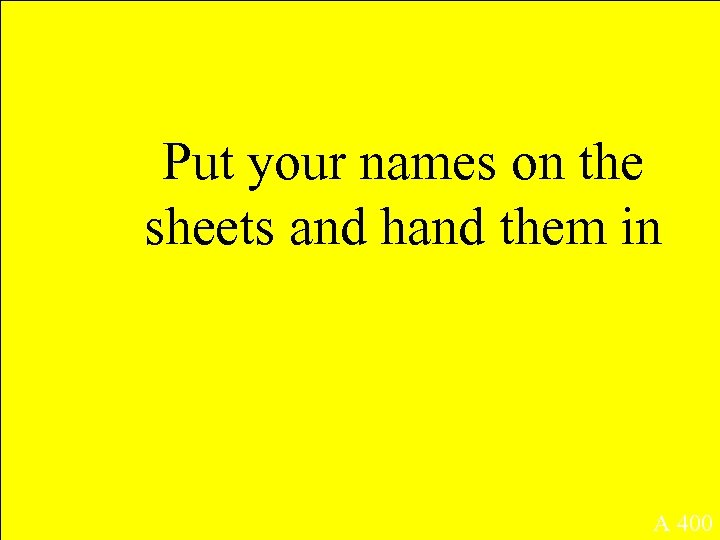Put your names on the sheets and hand them in A 400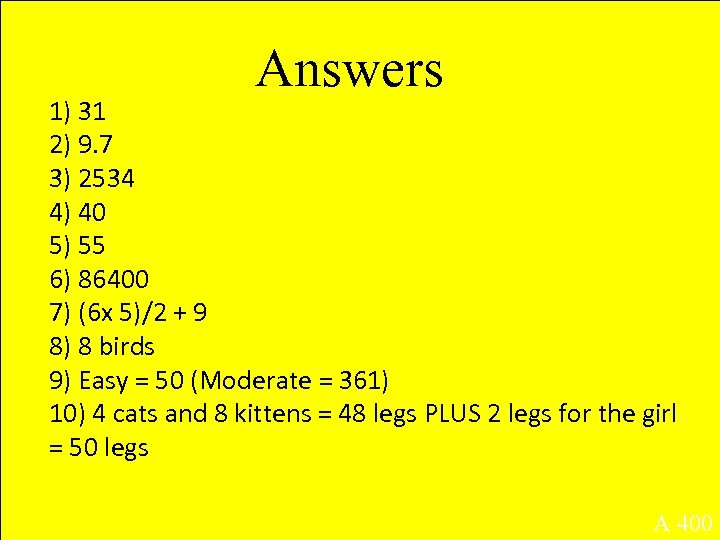Answers 1) 31 2) 9. 7 3) 2534 4) 40 5) 55 6) 86400 7) (6 x 5)/2 + 9 8) 8 birds 9) Easy = 50 (Moderate = 361) 10) 4 cats and 8 kittens = 48 legs PLUS 2 legs for the girl = 50 legs A 400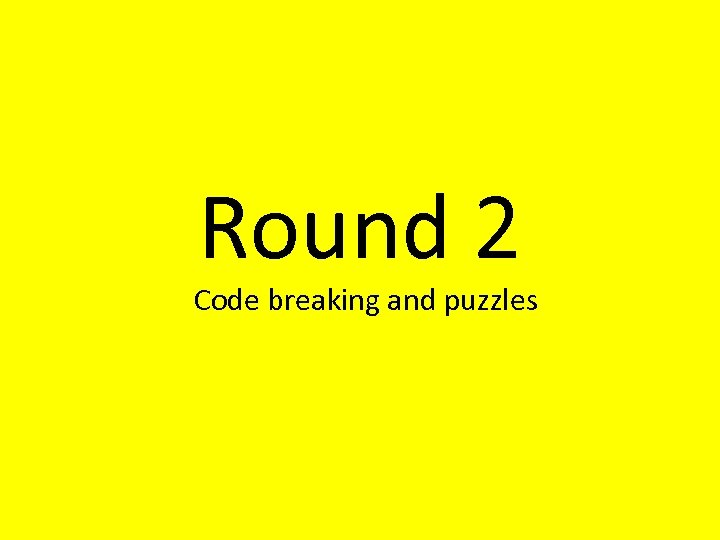Round 2 Code breaking and puzzles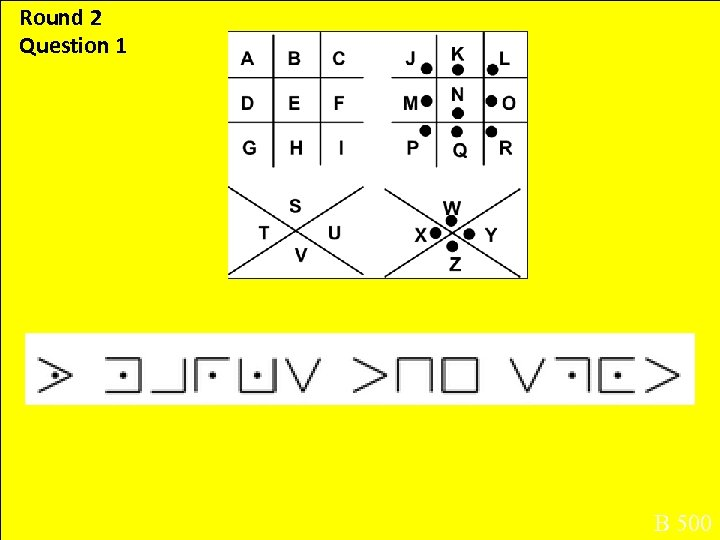Round 2 Question 1 B 500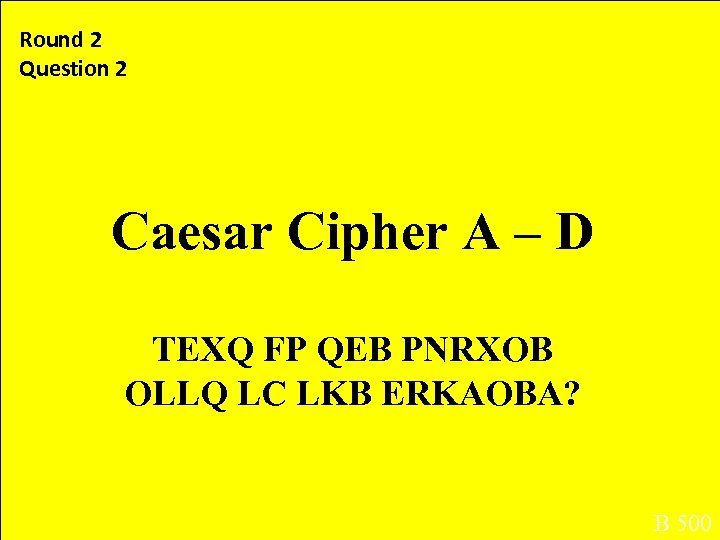Round 2 Question 2 Caesar Cipher A – D TEXQ FP QEB PNRXOB OLLQ LC LKB ERKAOBA? B 500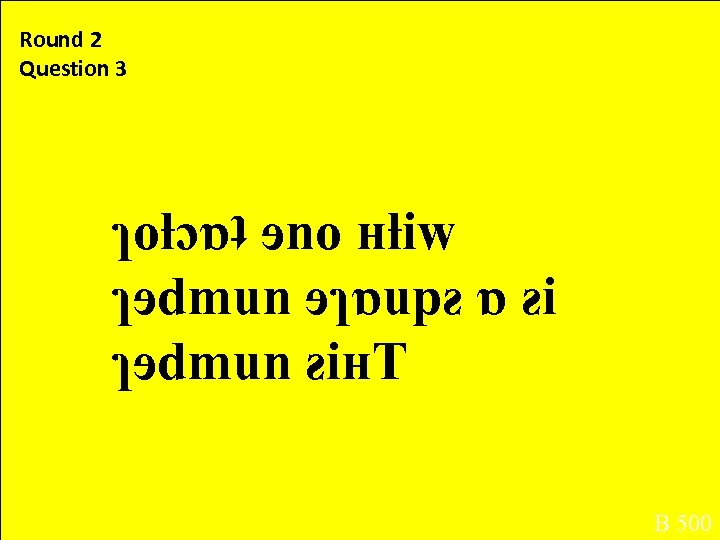Round 2 Question 3 ɿoƚɔɒʇ ɘno ʜƚiw ɿɘdmun ɘɿɒupƨ ɒ ƨi ɿɘdmun ƨiʜT B 500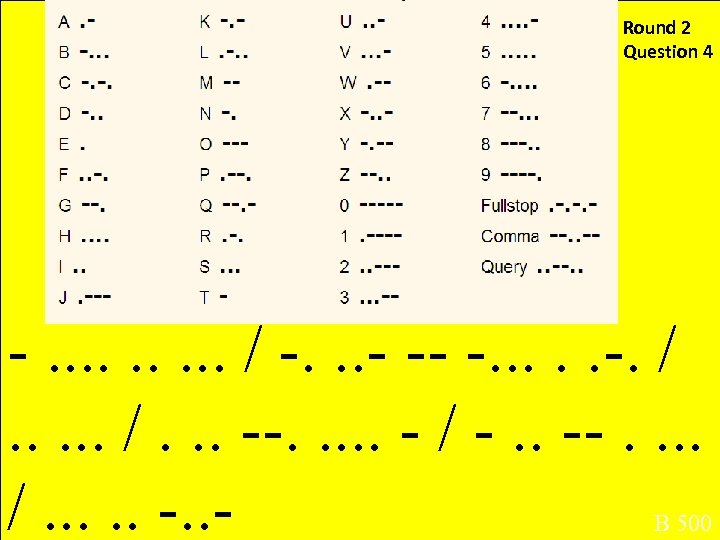Round 2 Question 4 -. . . . / -. . . - -- -. /. . . --. . - / -. . --. . . . /. . - B 500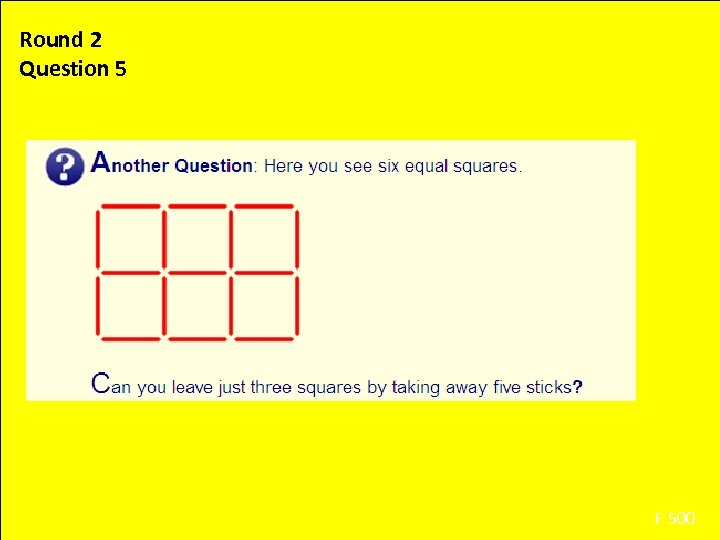Round 2 Question 5 F 500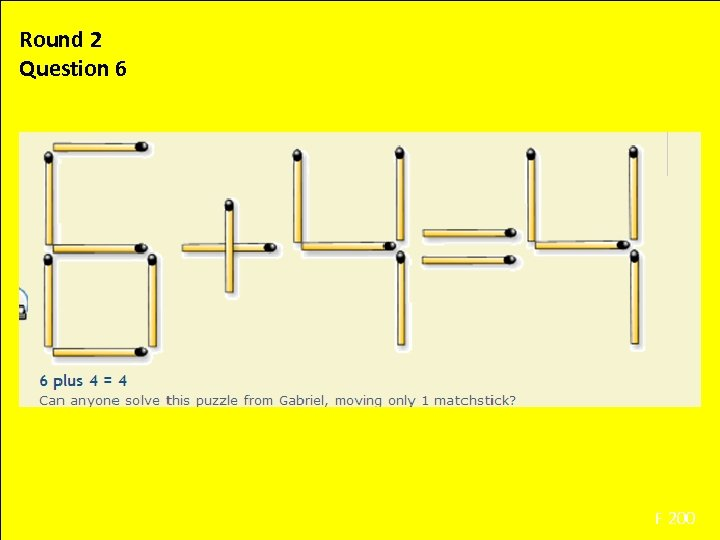Round 2 Question 6 F 200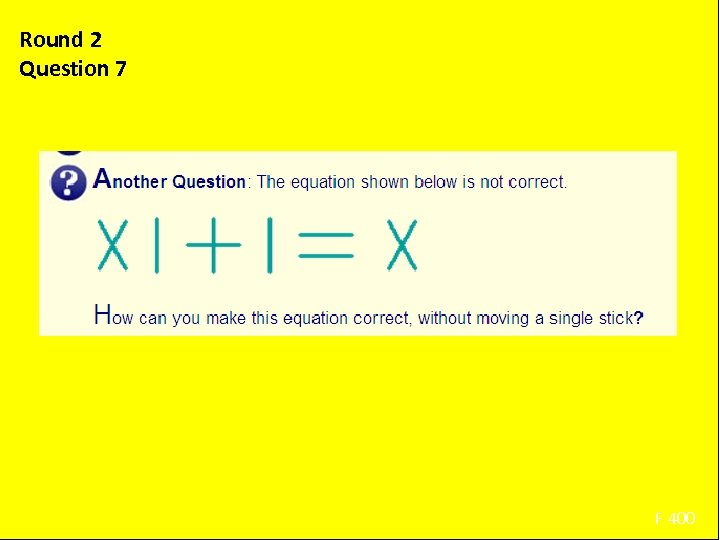Round 2 Question 7 Using only the arithmetic symbols and 2, 5, 6, 9, make 24 F 400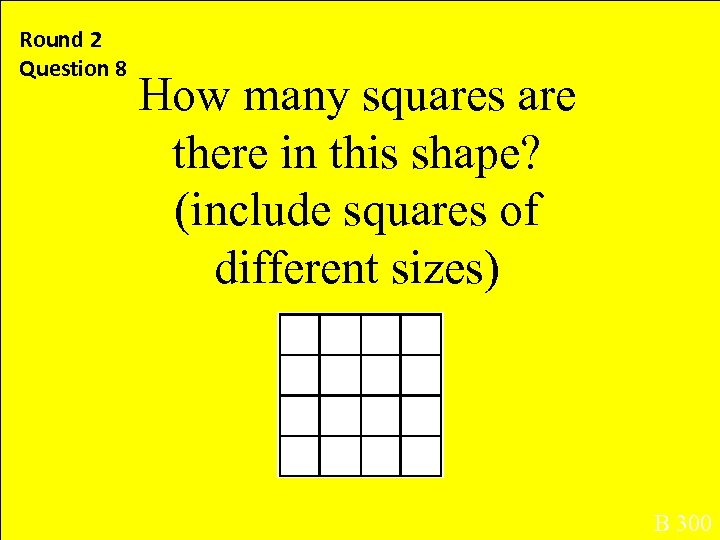Round 2 Question 8 How many squares are there in this shape? (include squares of different sizes) B 300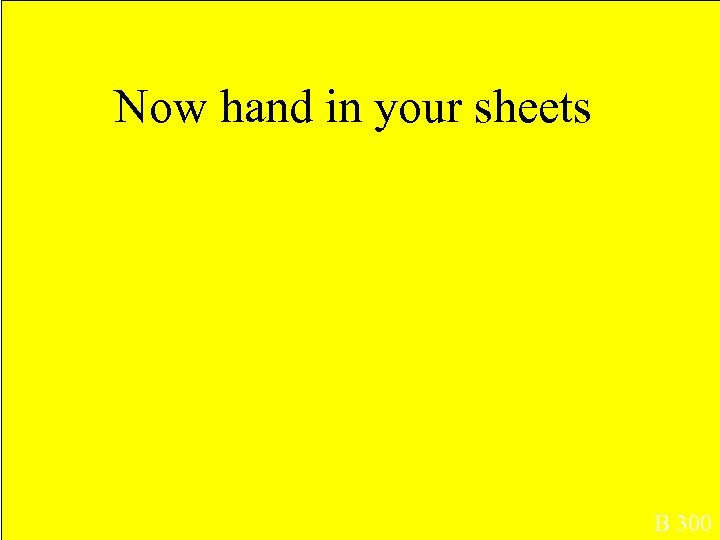Now hand in your sheets B 3002) 3) 4) 5) Answers 1) X marks the spot What is the square root of one hundred? (=10) This number is a square number with only 1 factor (= 1) This number is eight times six (=48) The shape looks like this: B 300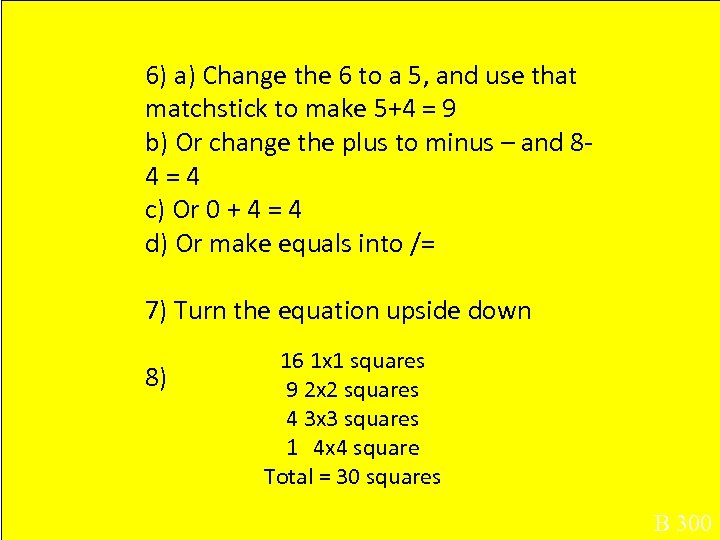6) a) Change the 6 to a 5, and use that matchstick to make 5+4 = 9 b) Or change the plus to minus – and 84=4 c) Or 0 + 4 = 4 d) Or make equals into /= 7) Turn the equation upside down 8) 16 1 x 1 squares 9 2 x 2 squares 4 3 x 3 squares 1 4 x 4 square Total = 30 squares B 300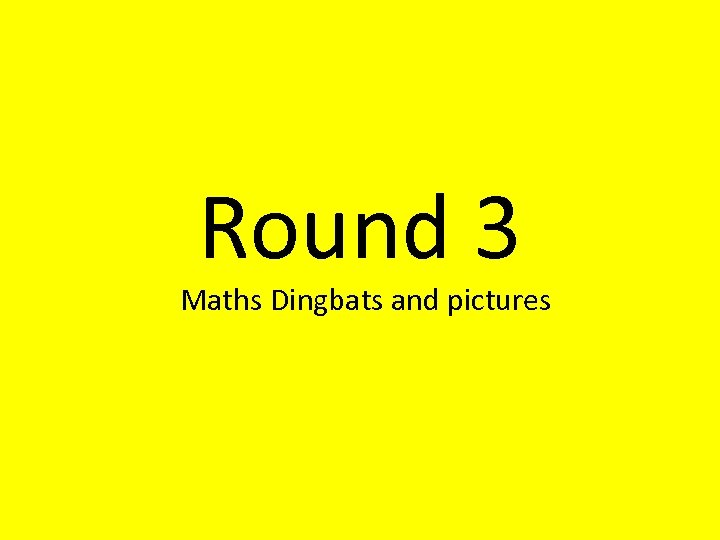Round 3 Maths Dingbats and pictures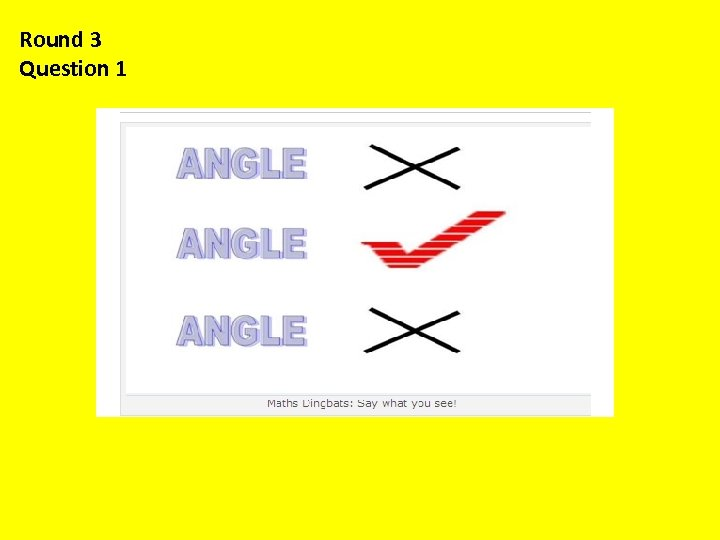Round 3 Question 1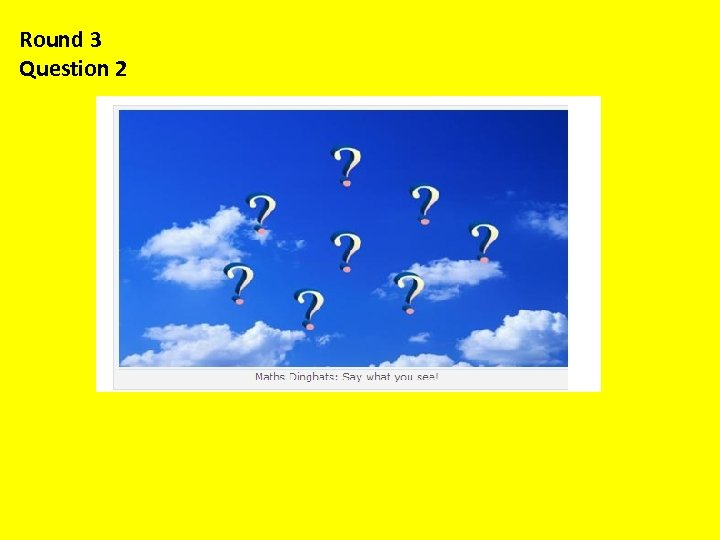Round 3 Question 2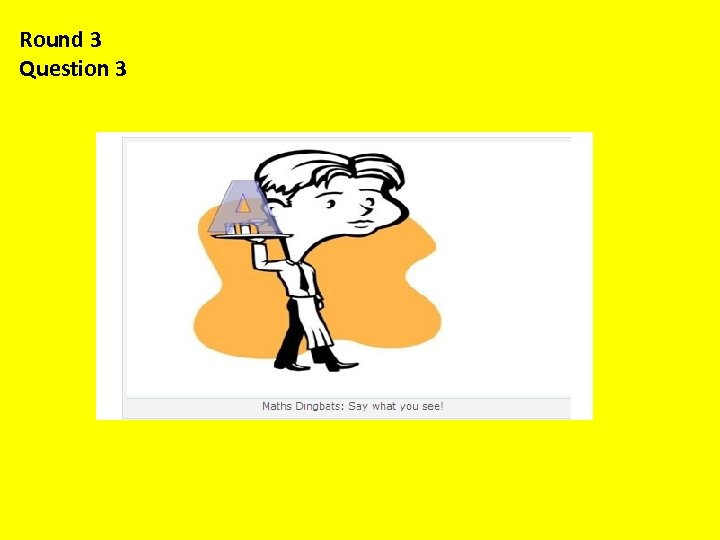Round 3 Question 3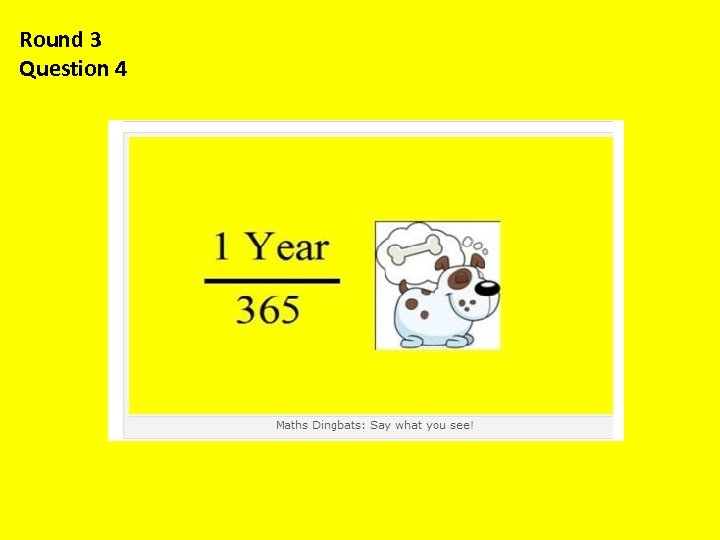Round 3 Question 4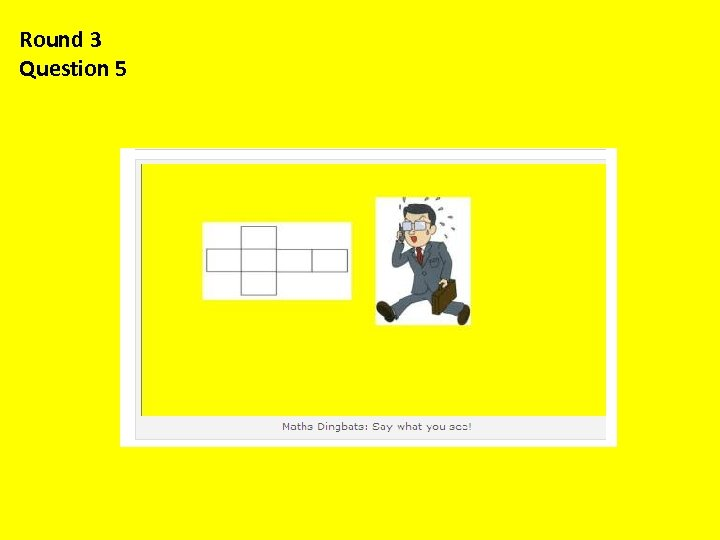Round 3 Question 5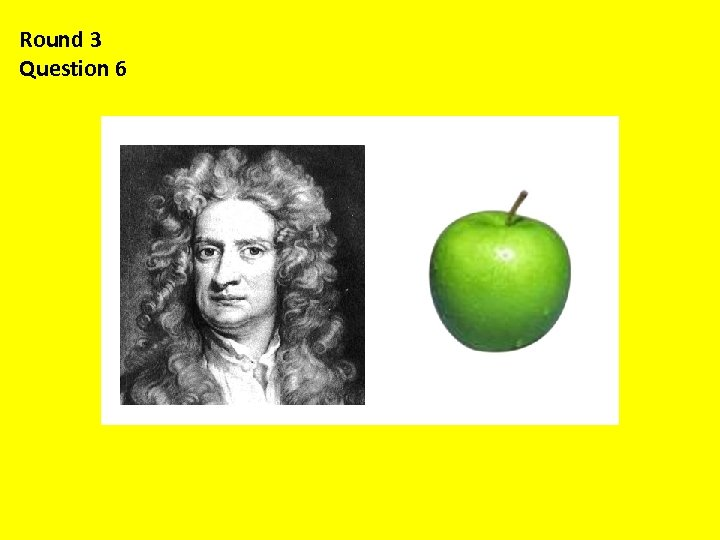Round 3 Question 6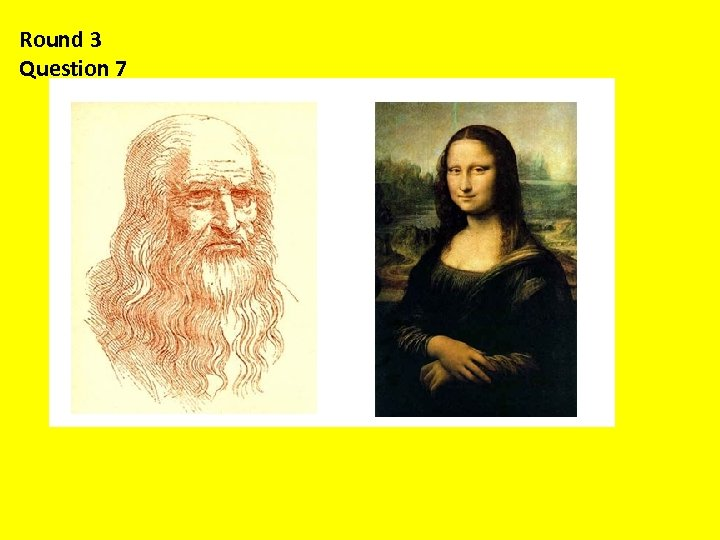Round 3 Question 7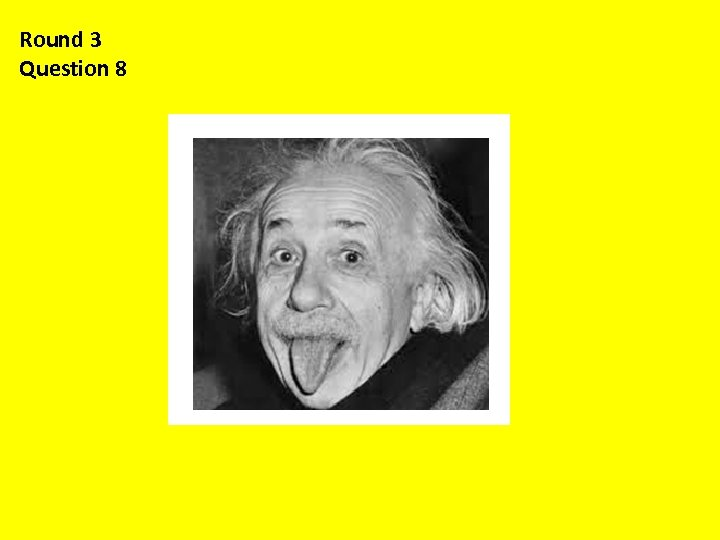Round 3 Question 8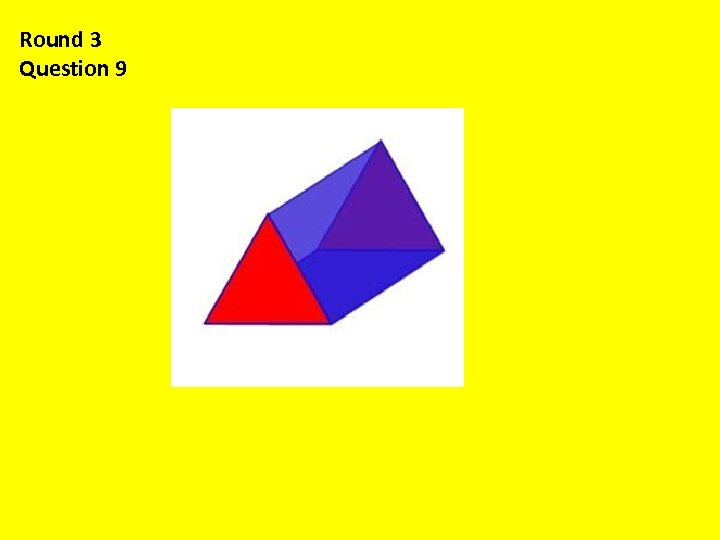Round 3 Question 9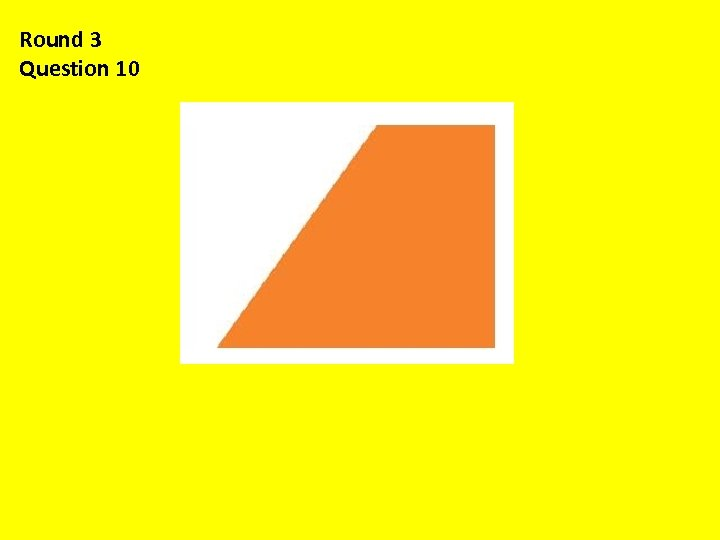Round 3 Question 10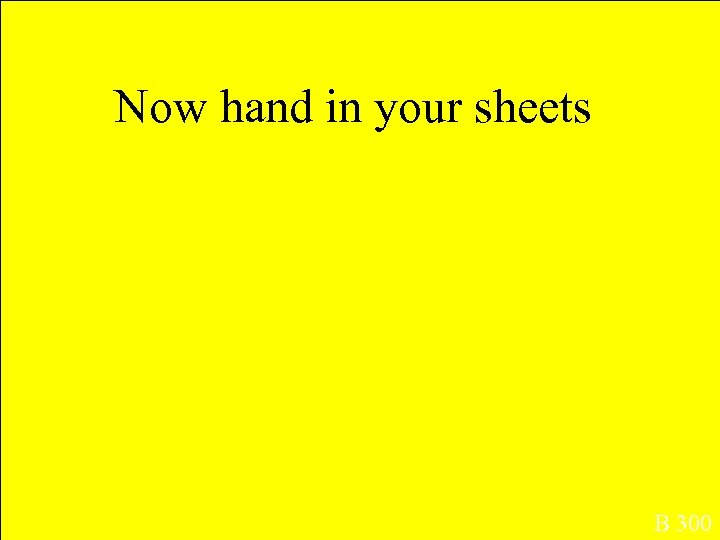Now hand in your sheets B 300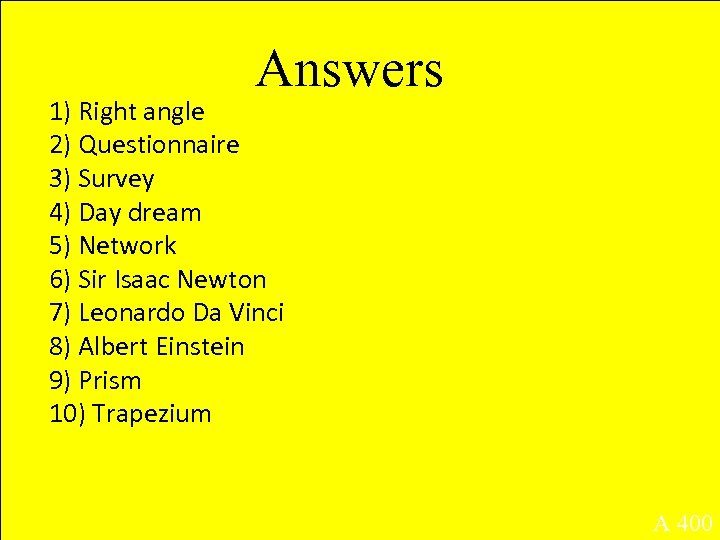Answers 1) Right angle 2) Questionnaire 3) Survey 4) Day dream 5) Network 6) Sir Isaac Newton 7) Leonardo Da Vinci 8) Albert Einstein 9) Prism 10) Trapezium A 400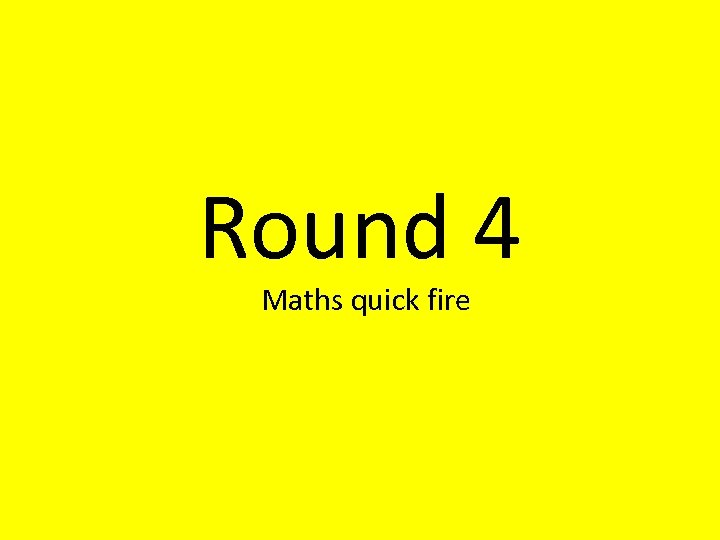Round 4 Maths quick fire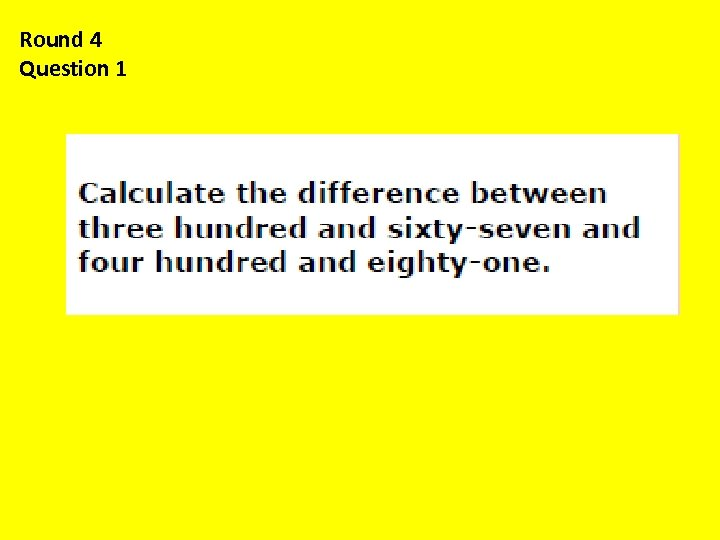Round 4 Question 1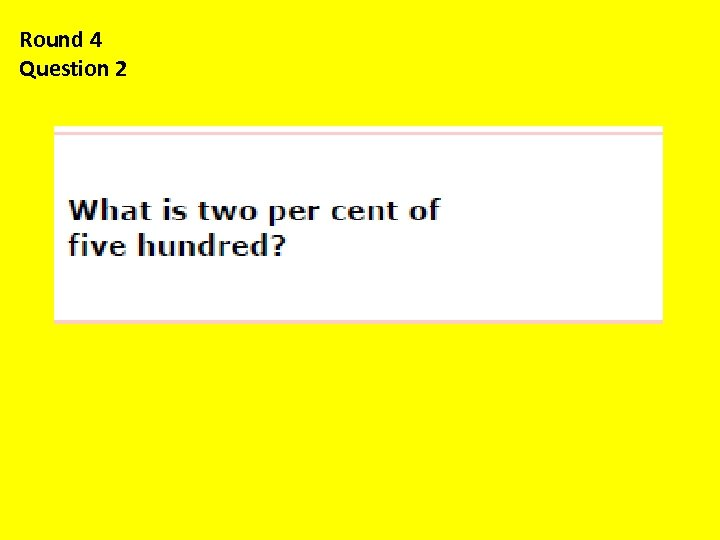Round 4 Question 2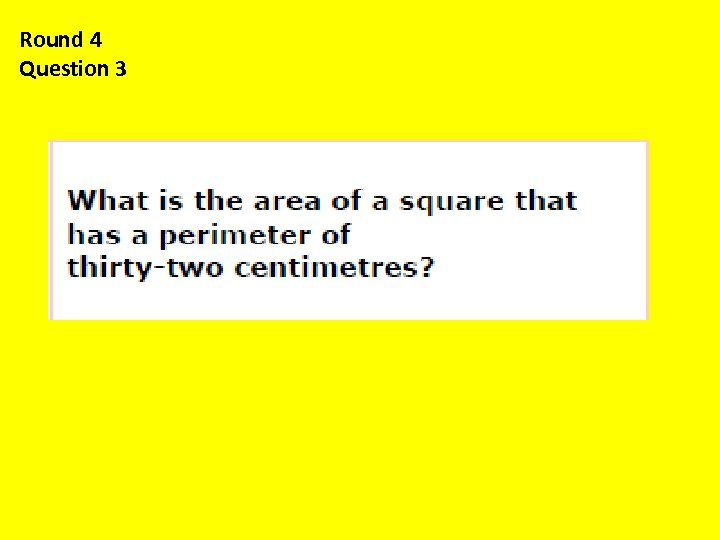Round 4 Question 3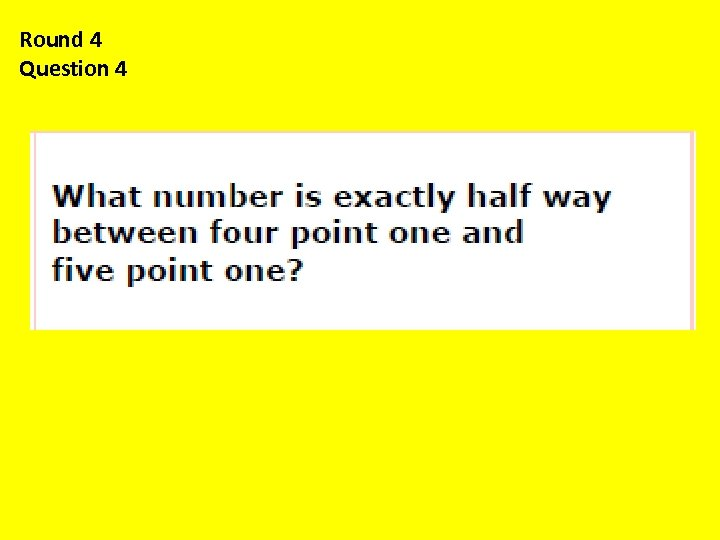Round 4 Question 4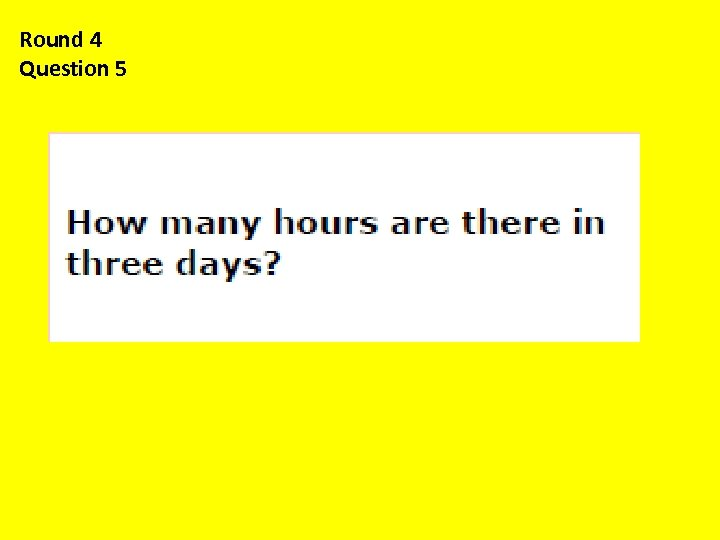Round 4 Question 5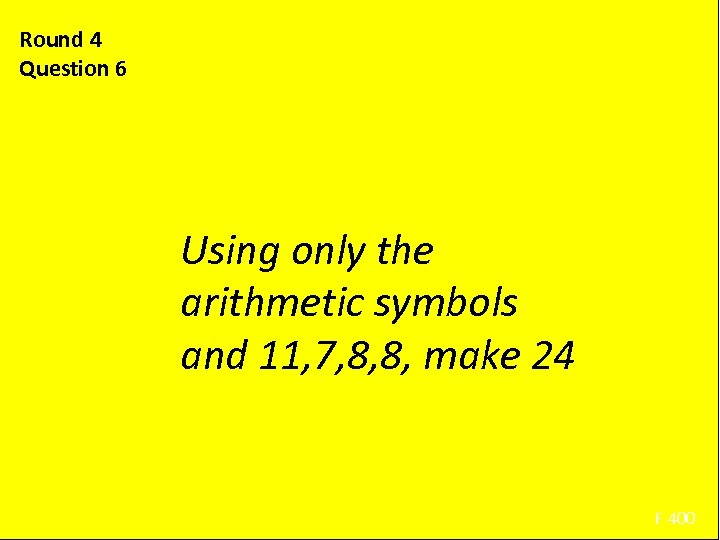Round 4 Question 6 Using only the arithmetic symbols and 11, 7, 8, 8, make 24 F 400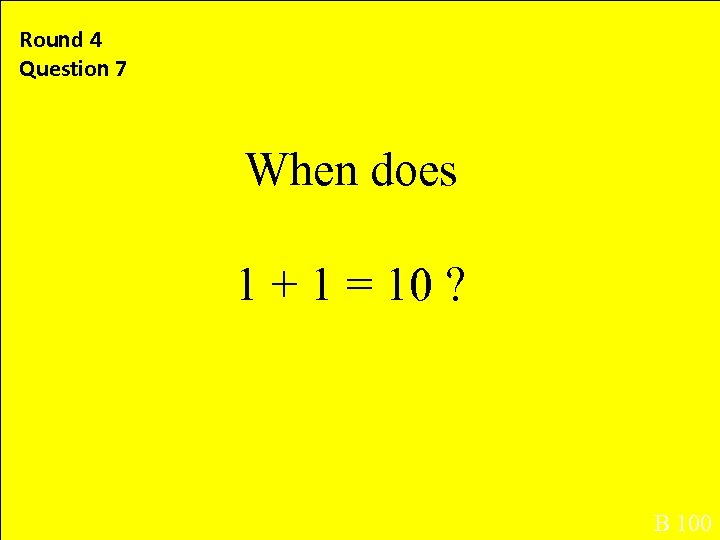Round 4 Question 7 When does 1 + 1 = 10 ? B 100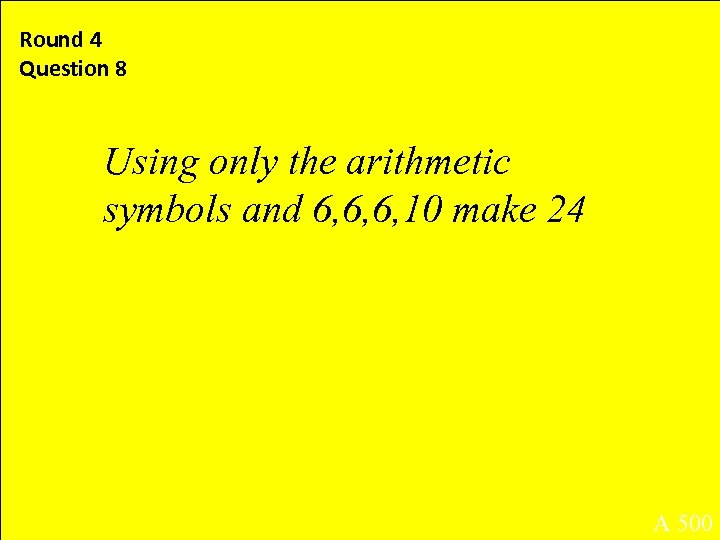Round 4 Question 8 Using only the arithmetic symbols and 6, 6, 6, 10 make 24 A 500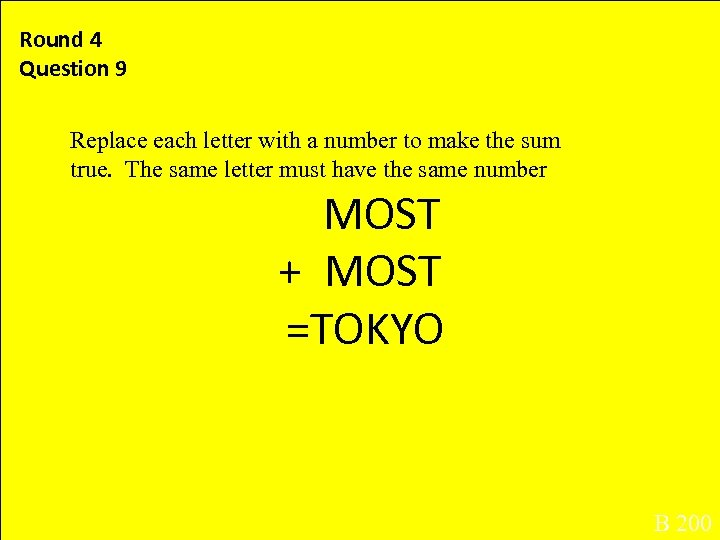Round 4 Question 9 . Replace each letter with a number to make the sum true. The same letter must have the same number MOST + MOST =TOKYO B 200Now hand in your sheets B 300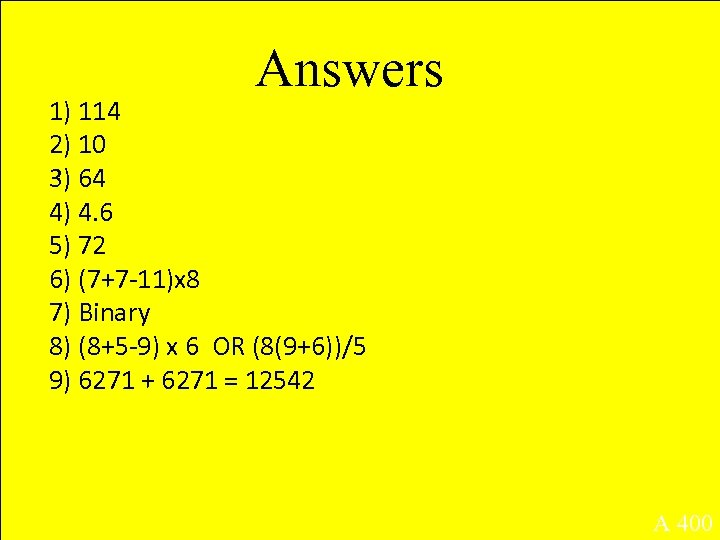Answers 1) 114 2) 10 3) 64 4) 4. 6 5) 72 6) (7+7 -11)x 8 7) Binary 8) (8+5 -9) x 6 OR (8(9+6))/5 9) 6271 + 6271 = 12542 A 400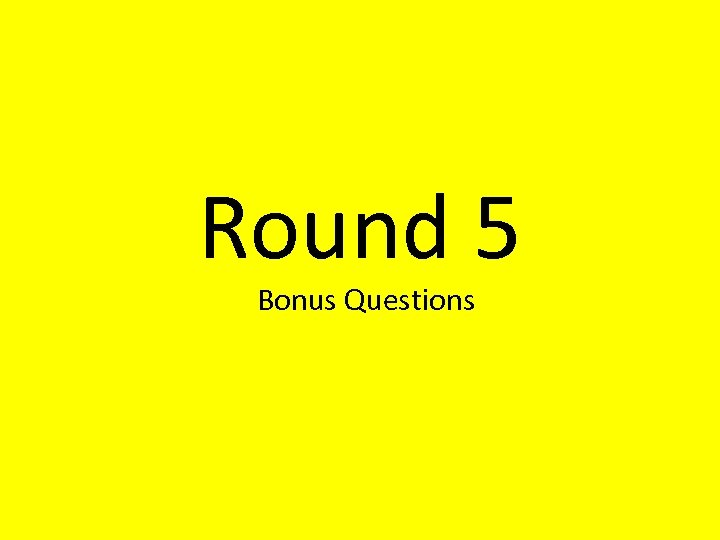Round 5 Bonus Questions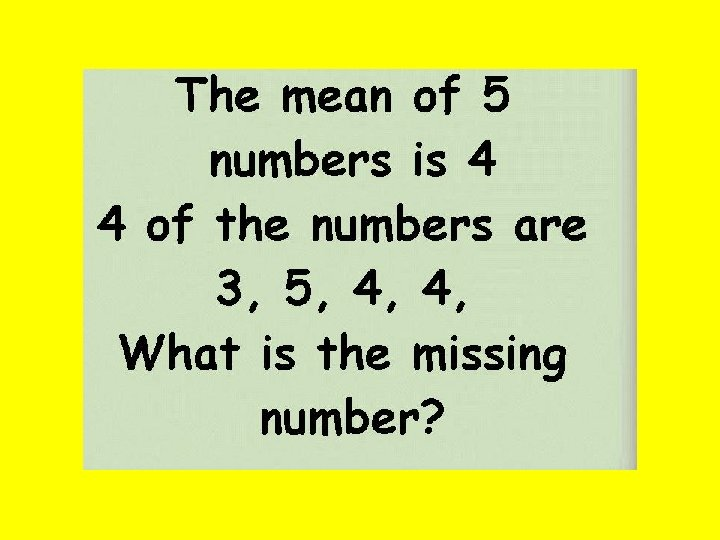Round 5 Bonus Questions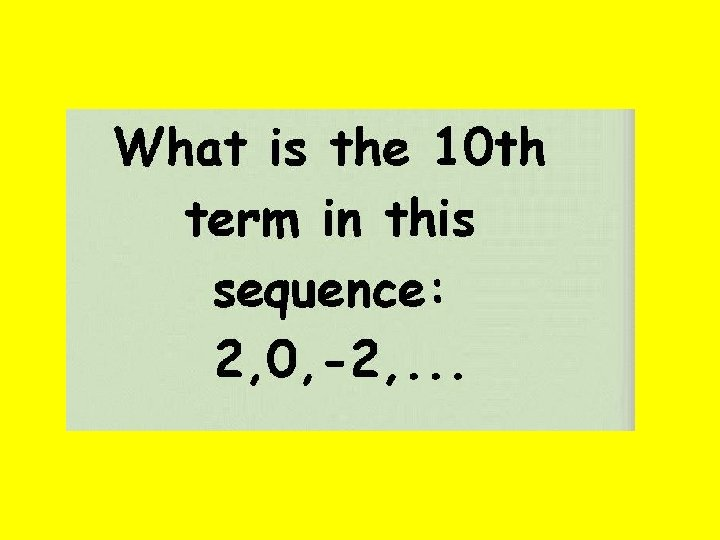Round 5 Bonus Questions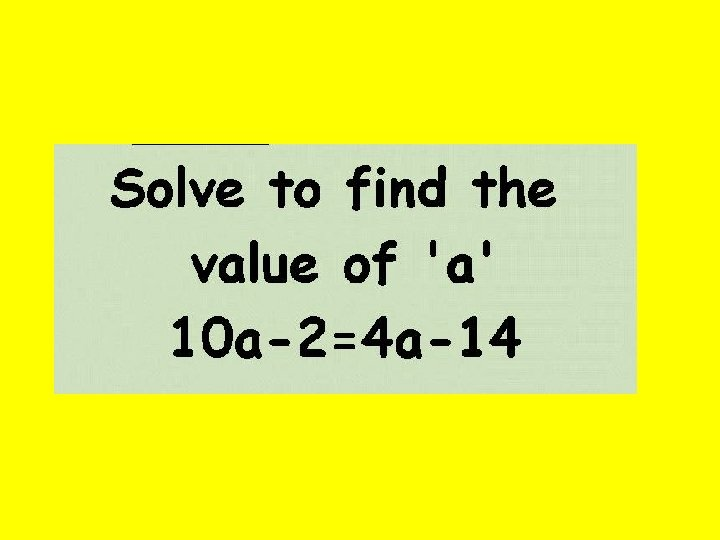Round 5 Bonus Questions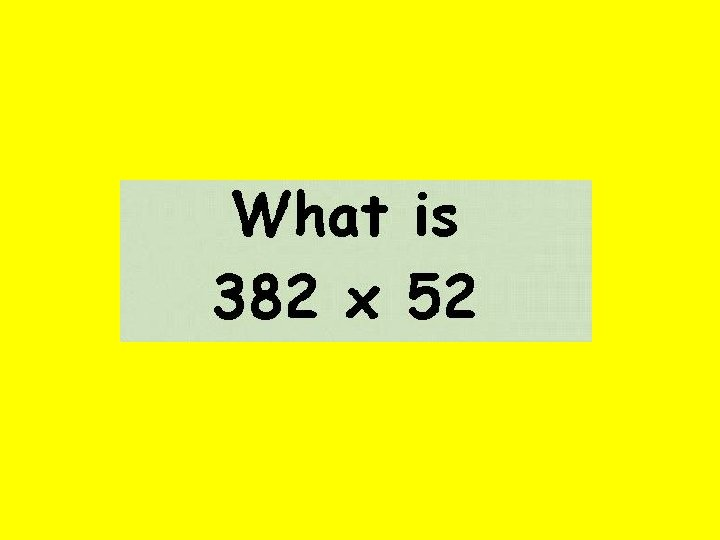Round 5 Bonus Questions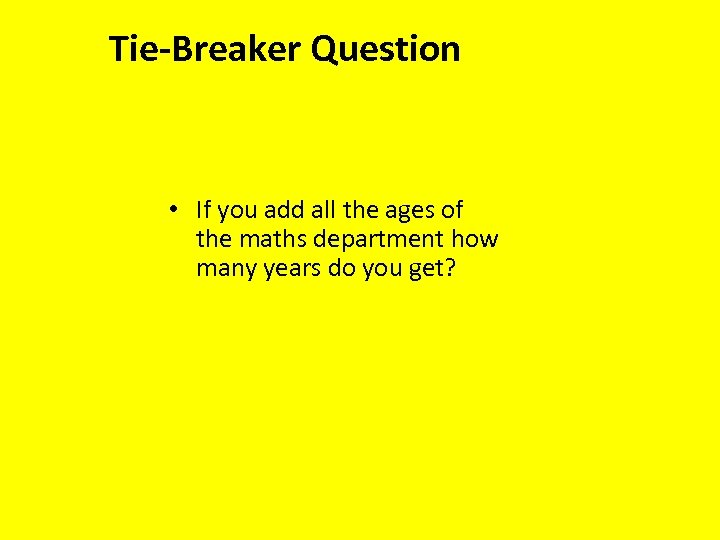Tie-Breaker Question • If you add all the ages of the maths department how many years do you get?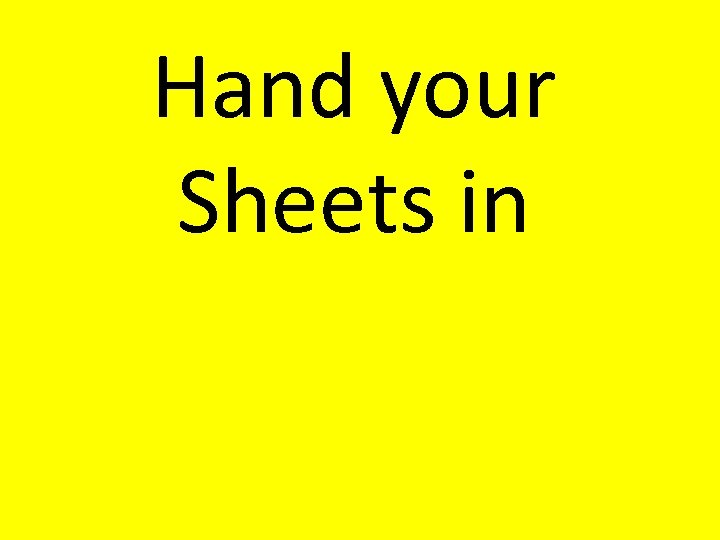Hand your Sheets in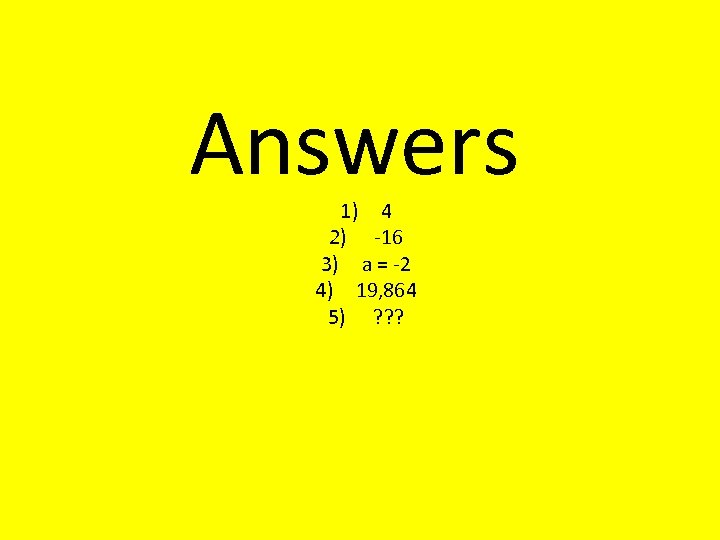Answers 1) 4 2) -16 3) a = -2 4) 19, 864 5) ? ? ?